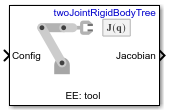# Get Jacobian

Geometric Jacobian for robot configuration

•Libraries:
Robotics System Toolbox / Manipulator Algorithms

## Description

The Get Jacobian block returns the geometric Jacobian relative to the base for the specified end effector at the given configuration of a `rigidBodyTree` robot model.

The Jacobian maps the joint-space velocity to the end-effector velocity relative to the base coordinate frame. The end-effector velocity equals:

``

ω is the angular velocity, υ is the linear velocity, andis the joint-space velocity.

## Ports

### Input

expand all

Robot configuration, specified as a vector of positions for all nonfixed joints in the robot model, as set by the Rigid body tree parameter. You can also generate this vector for a complex robot using the `homeConfiguration` or `randomConfiguration` functions inside a Constant or MATLAB Function block.

### Output

expand all

Geometric Jacobian of the end effector with the specified configuration, Config, returned as a 6-by-n matrix, where n is the number of degrees of freedom of the end effector. The Jacobian maps the joint-space velocity to the end-effector velocity relative to the base coordinate frame. The end-effector velocity equals:

``

ω is the angular velocity, υ is the linear velocity, andis the joint-space velocity.

## Parameters

expand all

Robot model, specified as a `rigidBodyTree` object. You can also import a robot model from an URDF (Unified Robot Description Format) file using `importrobot`.

The default robot model, `twoJointRigidBodyTree`, is a robot with revolute joints and two degrees of freedom.

End effector for `Jacobian`, specified as a body name from the Rigid body tree robot model. To access body names from the robot model, click .

• `Interpreted execution` — Simulate model using the MATLAB® interpreter. This option shortens startup time but has a slower simulation speed than ```Code generation```. In this mode, you can debug the source code of the block.

• `Code generation` — Simulate model using generated C code. The first time you run a simulation, Simulink® generates C code for the block. The C code is reused for subsequent simulations, as long as the model does not change. This option requires additional startup time, but the speed of the subsequent simulations is comparable to ```Interpreted execution```.

Tunable: No

## Version History

Introduced in R2018a